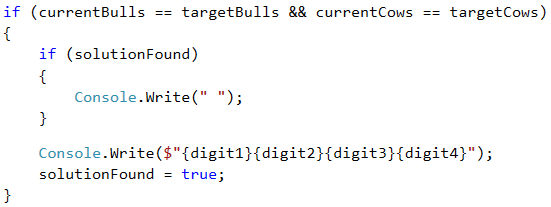# Problem: Bulls and Cows

We all know the game called "Bulls and Cows" (https://en.wikipedia.org/wiki/Bulls_and_cows). Upon having a particular 4-digit secret number and a 4-digit suggested number, the following rules are applied:

• If a digit in the suggested number matches a digit in the secret number and is located at the same position, we have a bull.
• If a digit in the suggested number matches a digit in the secret number, but is located at a different position, we have a cow.
Secret number 1 4 8 1 Comment
Suggested number 8 8 1 1 Bulls = 1
Cows = 2
Secret number 2 2 4 1 Comment
Suggested number 9 9 2 4 Bulls = 0
Cows = 2

Upon having a particular secret number and the bulls and cows pertaining to it, our task is to find all possible suggested numbers in ascending order.

If there are no suggested numbers that match the criteria provided from the console, we must print "No".

## Input Data

The input data is read from the console. The input consists of 3 text lines:

• The first line contains the secret number.
• The second line contains the number of bulls.
• The third line contains the number of cows.

The input data will always be valid. There is no need to verify them.

## Output Data

The output data must be printed on the console. The output must consist of a single line, holding all suggested numbers, space separated. If there are no suggested numbers that match the criteria provided from the console, we must print “No”.

## Constraints

• The secret number will always consist of 4 digits in the range [1..9].
• The number of cows and bulls will always be in the range [0..9].
• Allowed execution time: 0.15 seconds.
• Allowed memory: 16 MB.

## Sample Input and Output

Input Output
2228
2
1
1222 2122 2212 2232 2242 2252 2262 2272 2281 2283 2284 2285 2286 2287 2289 2292 2322 2422 2522 2622 2722 2821 2823 2824 2825 2826 2827 2829 2922 3222 4222 5222 6222 7222 8221 8223 8224 8225 8226 8227 8229 9222
Input Output
1234
3
0
1134 1214 1224 1231 1232 1233 1235 1236 1237 1238 1239 1244 1254 1264 1274 1284 1294 1334 1434 1534 1634 1734 1834 1934 2234 3234 4234 5234 6234 7234 8234 9234
Input Output
1234
3
1
No

## Hints and Guidelines

We will solve the problem in a few steps:

• We will read the input data.
• We will generate all possible four-digit combinations (candidates for verification).
• For each generated combination we will calculate how many bulls and how many cows it has according to the secret number. Upon matching the needed bulls and cows, we will print the combination.

We have 3 lines in the input data:

• Secret number.
• Number of desired bulls.
• Number of desired cows.

Reading the input data is trivial: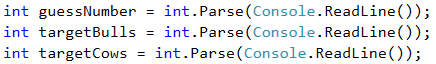### Declaring a Flag

Before starting to write the algorithm for solving our problem, we must declare a flag that indicates whether a solution is found:If after finishing our algorithm this flag is still false, then we will print No on the console, as specified in the requirements.### Generating Four-Digit Numbers

Let's start analyzing our problem. What we need to do is analyze all numbers from 1111 to 9999, excluding those that contain zeroes (for example 9011, 3401, etc. are invalid). What is the easiest way to generate all these numbers? We will use nested loops. As we have a 4-digit number, we will have 4 nested loops, as each of them will generate an individual digit in our number for testing.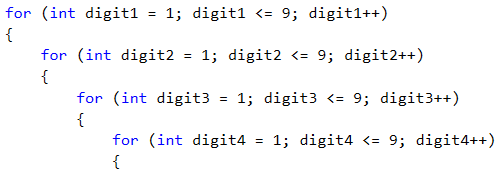Thanks to these loops, we have access to every digit of all numbers that we need to check. Our next step is to separate the secret number into digits. This can be achieved very easily using a combination of integer division and modular division.Only two last steps remain until we start analyzing how many cows and bulls there are in a particular number. Accordingly, the first one is the declaration of counter variables in the nested loops, in order to count the cows and bulls for the current number. The second step is to make copies of the digits of the current number that we will analyze, in order to prevent problems upon working with nested loops, in case we make changes to them.We are ready to start analyzing the generated numbers.

### Counting the Bulls

What logic can we use? The easiest way to check how many cows and bulls there are inside a number is via a sequence of if-else conditions. Yes, this is not the most optimal way, but in order to stick to what is covered in the current book, we will use this approach.

What conditions do we need?

The condition for the bulls is very simple – we check whether the first digit of the generated number matches the same digit in the secret number. We remove the digits that are already checked in order to avoid repetitions of bulls and cows.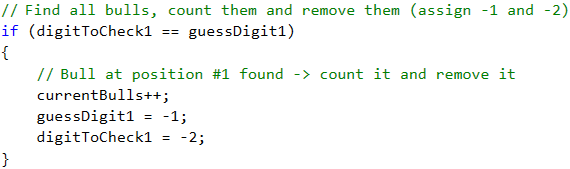We repeat the action for the second, third and fourth digit.

### Counting the Cows

We will apply the following condition for the cows – first we will check whether the first digit of the generated number matches the second one, the third one or the fourth digit of the secret number. An example for the implementation: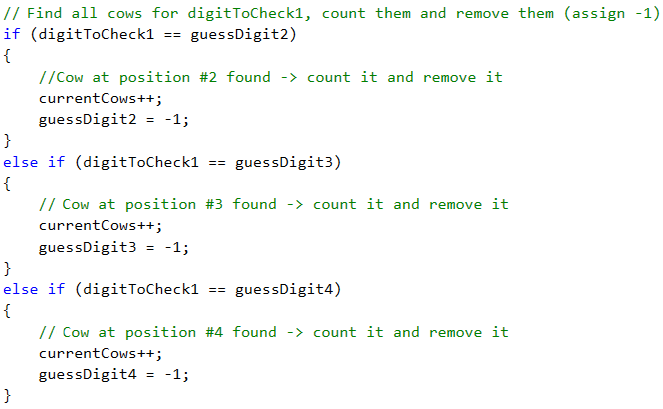After that, we sequentially check whether the second digit of the generated number matches the first one, the third one or the fourth digit of the secret number; whether the third digit of the generated number matches the first one, the second one or the fourth digit of the secret number; and finally, we check whether the fourth digit of the generated number matches the first one, the second one or the third digit of the secret number.

### Printing the Output

After completing all conditions, we just need to check whether the bulls and cows in the currently generated number match the desired bulls and cows read from the console. If this is true, we print the current number on the console.